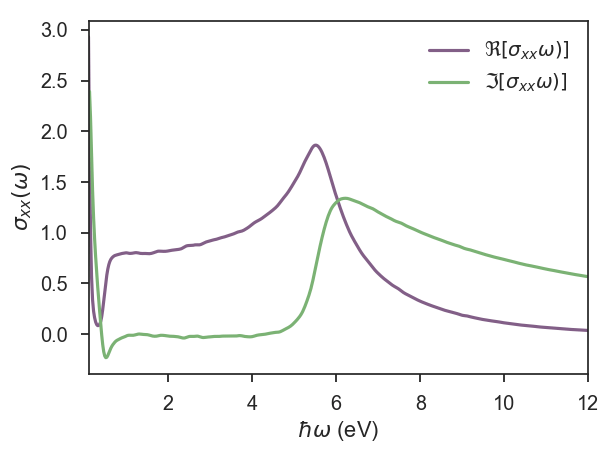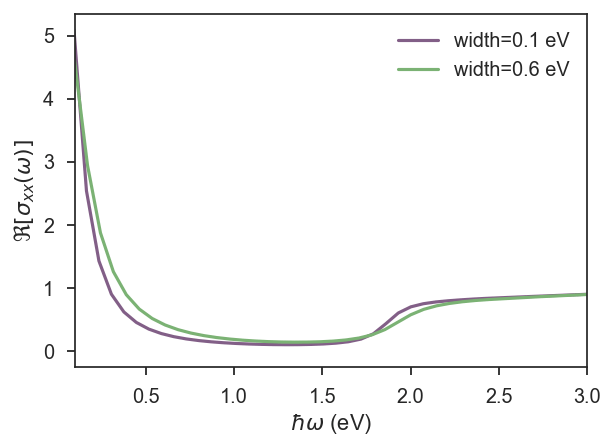# Optical Conductivity: Graphene with Gaussian Disorder

KITE also calculates the optical conductivity of a given lattice for a given Fermi energy. To illustrate this capability, we calculate the optical conductivity of disordered graphene, that can be compared qualitativelly with previous results 1.

Instead of defining the lattice in our python script, we can use of one the pre-defined lattices from pybinding:

from pybinding.repository import graphene

lattice = graphene.monolayer()


To illustrate a different type of disorder, we random on-site energies that follow a Gaussian distribution

disorder = kite.Disorder(lattice)


where we define the type of statistical distribution, the sublattices they are located, the mean value of the distribution and its width.

After configuring the system, as presented in section getting started, it is time to set the calculation:

calculation = kite.Calculation(configuration)

calculation.conductivity_optical(num_points=1000, num_disorder=1,
num_random=20, num_moments=512, direction='xx')


The optical conductivity can also be calculated in different directions, which can be quite interesting in the case of Hamiltonians with non-trivial topology that also present transverse optical conductivity. However, in this example we focus on longitudinal optical conductivity. The other quantities that can be set in the python script are the same of the density of states: number of energy poins used in KITE-tools, moments in the expansion, random vectors and disorder realisations.

When calculating the optical conductivity, it is necessary to pass the Fermi energy as a parameter to the executable KITE-tools. Run ./KITE-tools –help for syntax examples.

The results of the real and imaginary parts of the optical conductivity presented in the first figure were obtained on a normal desktop with calculations that took 8 minutes for a system with $N=512\times 512$ units cells and 512 expansion moments.For systems sizes of $N=1536\times 1536$ unit cells and two different Gaussian widths, we show $\Re [\sigma_{xx}(\omega)]$ for low frequencies, where we can see Drude’s peak for $\omega\rightarrow 0$ and the onset of interband transitions at $\hbar \omega>2 E_F$2.The complete python script for this calculation can be found here

1 Shengjun Yuan, Rafael Roldán, Hans De Raedt, Mikhail I. Katsnelson, Phys. Rev. B 84, 195418 (2011).

2 T. Stauber, N. M. R. Peres, A. K. Geim, Phys. Rev. B 78, 085432 (2008).